• Save

# Data Interpretation Question and Answer

Vikram Singh6 months ago 1.8K Views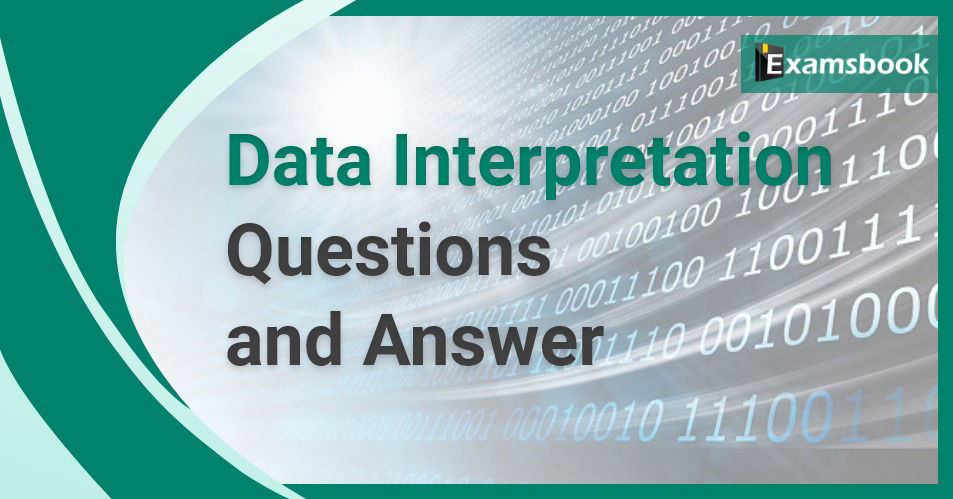Data Interpretation is an essential part of the Mathematics subject of Quantitative Aptitude. Basically, data interpretation is based on the bar graph, line graph, tabulation,s and pie chart questions. Here I am sharing some important questions related to this topic.

With the help of these questions, you can easily understand the topic of Data Interpretation and improve your performance.

## Selective Data Interpretation Questions

Study the given graph and answer the question that follows.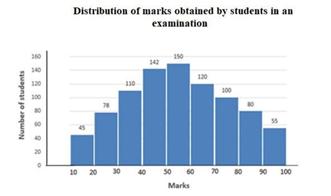Q :

If 60% of the total number of students obtaining 50 marks or more but less than 70 marks are girls and 55 % of the total number of students obtaining 70 marks or more but less than 90 marks are also girls. Then the total number of girls obtaining 50 marks or more but less than 90 marks is:

(A) 261

(B) 207

(C) 236

(D) 257

Correct Answer : A

Study the given graph and answer the question that follows.Q :

The total number of students obtaining less than 50 marks is what percentage more than the total number of students obtaining 60 marks or more ( correct to one decimal place).

(A) 6.8

(B) 6.2

(C) 4.4

(D) 5.6

Correct Answer : D

Study the given pie chart and answer the question that follows.
Distribution (Degree wise) of students studying in schools A, B, C, D and E
Total Number of students =9000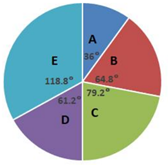Q :

If the ratio of the number of boys to that of girls in school C is 6 : 5 and that in school B is 7 : 11,then what is the ratio of the number of boys in school C to the number of girls in school B?

(A) 10 : 11

(B) 11 : 10

(C) 12 : 11

(D) 11: 12

Correct Answer : C

Study the given pie chart and answer the question that follows.
Distribution (Degree wise) of students studying in schools A, B, C, D and E
Total Number of students =9000Q :

The number of students in School E exceeds the total number of students in school A and B by x, where x lies between:

(A) 440 and 460

(B) 420 and 440

(C) 460 and 480

(D) 400 and 420

Correct Answer : A

Study the given pie chart and answer the question that follows.
Distribution (Degree wise) of students studying in schools A, B, C, D and E
Total Number of students =9000Q :

The total number of students in schools D and E is what percentage more than the total number of students in school B and C?

(A) 25

(B) 20

(C) 30

(D) 35

Correct Answer : A

Directions: The following table represents the percentage marks of four students in six subjects.
Consider the table and answer questions based on it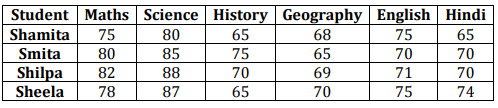Q :

The average marks obtained by the students in Geography and History are

(A) 68.75 and 68

(B) 70.5 and 69

(C) 68 and 68.75

(D) 68.75 and 68.5

Correct Answer : C

Directions: The following table represents the percentage marks of four students in six subjects.
Consider the table and answer questions based on itQ :

Average marks obtained in Science is higher than that of Maths by

(A) 1.5

(B) 20

(C) 6.25

(D) 40

Correct Answer : C

Directions: The following table represents the percentage marks of four students in six subjects.
Consider the table and answer questions based on itQ :

Who has the highest total marks?

(A) Shamita

(B) Smita

(C) Shilpa

(D) Sheela

Correct Answer : C

The given table represents the number of engineers recruited by four companies A, B, C and D over the years. Study the table carefully and answer the question that follow.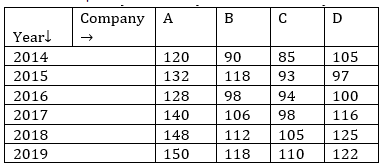Q :

The total number of engineers recruited by company A in 2014 to 2017 is what percentage more than the total number of engineers recruited by all four companies in 2019?

(A) 3.5

(B) 2.5

(C) 3

(D) 4

Correct Answer : D

Study the given graph and answer the question that follows.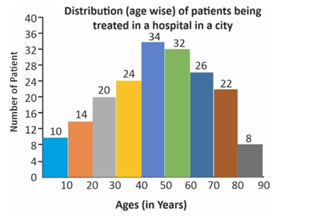Q :

The number of patients aged 10 or more years but below 40 years is what per cent less than the number of patients aged 50 or more years but below 80 years?

(A) 30.2

(B) 25

(C) 34

(D) 27.5

Correct Answer : D

### Vikram Singh

Providing knowledgable questions of Reasoning and Aptitude for the competitive exams.